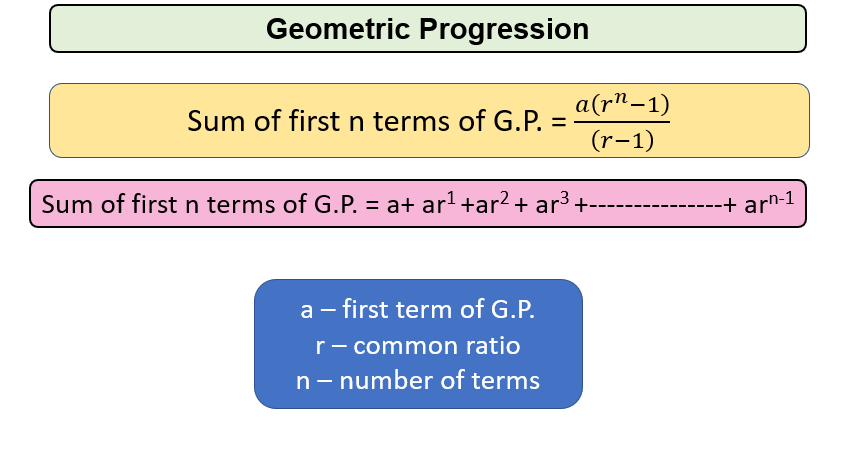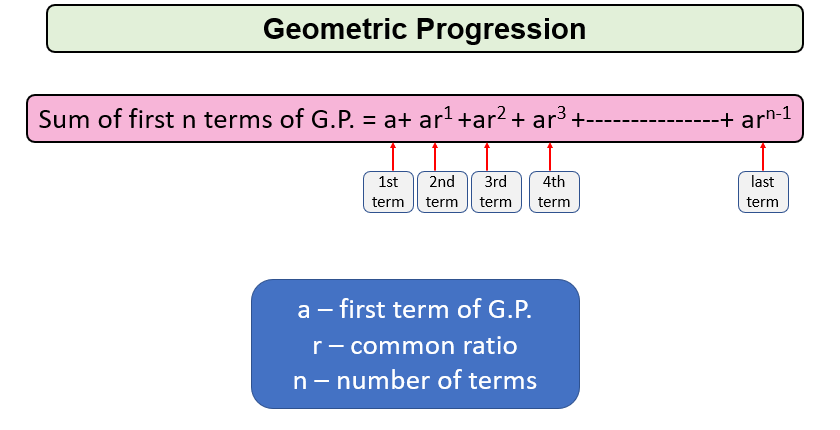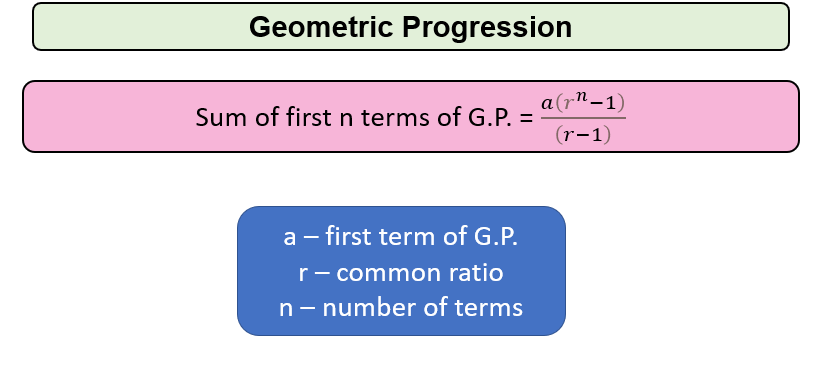# Program to find Sum of GP Series

Problem Statement: Given a geometric Progression (G.P) sequence with some inputs as:-

• a, first term
• r, common ratio
• n, number of terms

Write a program to find the sum of the geometric Progression Series.

Examples:

```Example 1:
Input: a=1 , r=0.5 , n=3
Output: 1.75
Explanation: The sum of given GP Series is 1.75

Example 2:
Input: a=3 , r=5 , n=2
Output: 18
Explanation: The sum of the given GP Series is 18
```

### Solution

Disclaimer: Don’t jump directly to the solution, try it out yourself first.

Let us understand what is Geometric Progression (G.P.)Solution 1:

Approach:  Just run the iterations one by one and add terms of G.P.For Eg.

a= 2, r=2, n=4

Initially , sum=0

First Term , a=2 , sum=2

Second Term , ar=4 , sum=6

Third Term , ar2=8, sum=14

Fourth / Last Term , ar3=16 , sum=30

Finally , sum is 30

Code:

## C++ Code

``````#include <iostream>
using namespace std;
// Function to find sum of G.P. series
float SumofGP(float a, float r, int n)
{
float sum = 0;
for (int i = 0; i < n; i++)
{
sum = sum + a;
a = a * r;
}
return sum;
}
int main()
{
float a = 2;     // First Term of G.P.
float r = 1.5; // Common Ration of G.P.
int n = 4;     // Number of terms in a G.P.
cout << "Sum of GP Series is "<<SumofGP(a, r, n);
return 0;
}
``````

Output: Sum of GP Series is 16.25

Time Complexity: O(n) since total number of iterations required are number of terms

Space Complexity: O(1)

## Java Code

``````import java.util.*;
public class Main {
public static double SumofGP(double a, double r, int n) {
double sum = 0.0;
for (int i = 0; i < n; i++) {
sum += a;
a = a * r;
}
return sum;
}
public static void main(String args[]) {
double a = 2.0; // First term of G.P.
double r = 1.5; // common ratio of G.P.
int n = 4; // Number of terms of G.P.
System.out.println("Sum of GP Series is "+SumofGP(a, r, n));
}
}
``````

Output: Sum of GP Series is 16.25

Time Complexity: O(n) since total number of iterations required are number of terms

Space Complexity: O(1)

Solution 2:

Approach:  Just apply the formula of the sum of G.P. SeriesFor Eg.

a= 2, r=2, n=4

sum= 2(24-1)/(2-1) =30

Finally, the sum is 30

Code:

## C++ Code

``````#include <iostream>
#include <math.h>
using namespace std;
// Function to find sum of G.P. series
float SumofGP(float a, float r, int n)
{
float sum = a * (pow(r, n) - 1) / (r - 1);
return sum;
}
int main()
{
float a = 2; // First Term of G.P.
float r = 2; // Common Ration of G.P.
int n = 4;   // Number of terms in a G.P.
cout << "Sum of GP Series is "<<SumofGP(a, r, n);
return 0;
}
``````

Output: Sum of GP Series is 30

Time Complexity: O(1) since direct formula is applied.

Space Complexity: O(1)

## Java Code

``````import java.util.*;
public class Main {
public static float SumofGP(float a, float r, int n) {
float sum = a * ((float) Math.pow(r, n) - 1) / (r - 1);
return sum;
}

public static void main(String args[]) {
float a = 2; // First term of G.P.
float r = 2; // common ratio of G.P.
int n = 4; // Number of terms of G.P.
System.out.println("Sum of GP Series is "+SumofGP(a, r, n));
}
}
``````

Output: Sum of GP Series is 30.0

Time Complexity: O(1) since direct formula is applied.

Space Complexity: O(1)

Special thanks to Gurmeet Singh for contributing to this article on takeUforward. If you also wish to share your knowledge with the takeUforward fam, please check out this article